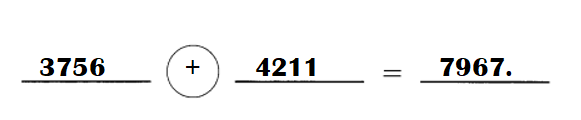# Math in Focus Grade 3 Chapter 5 Practice 2 Answer Key Real-World Problems: Addition and Subtraction

Go through the Math in Focus Grade 3 Workbook Answer Key Chapter 5 Practice 2 Real-World Problems: Addition and Subtraction to finish your assignments.

## Math in Focus Grade 3 Chapter 5 Practice 2 Answer Key Real-World Problems: Addition and Subtraction

Example
Janice has 1,458 stamps.
She has 396 fewer stamps than Ben.

a. How many stamps does Ben have?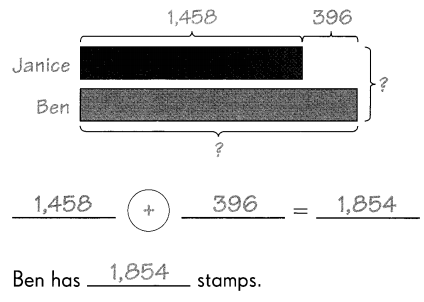b. How many stamps do they have in all?
1,458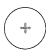1,854 = 3,312
They have 3,312 stamps in all.

Question 1.
There are 1,287 men at a baseball game.
There are 879 fewer women than men at the game.
a. How many women are at the game?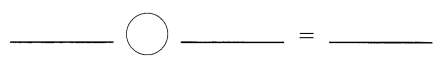____ women are at the game.
408 women are at the game.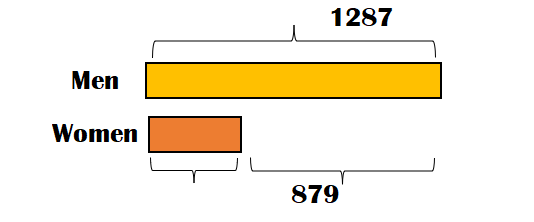Explanation:
Number of men at a baseball game = 1287.
There are 879 fewer women than men at the game.
Number of women at a baseball game = Number of men at a baseball game – 879
= 1287 – 879
= 408.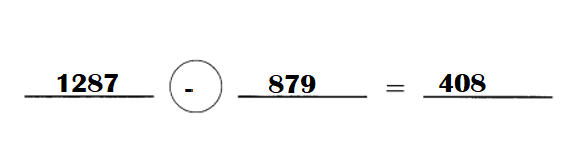b. How many adult spectators are at the game?____ adult spectators are at the game.
Number of adult spectators are at the game = 879.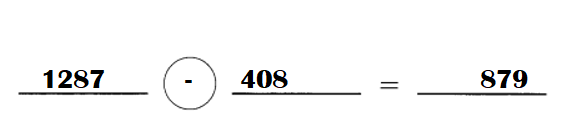Explanation:
Number of men at a baseball game = 1287.
Number of women at a baseball game = 408.
Number of adult spectators are at the game = Number of men at a baseball game – Number of women at a baseball game
= 1287 – 408
= 879.

Question 2.
A school sets aside $4,756 for its athletic fund. It sets aside$1,297 less for its library fund.

a. How much money is in the library fund?$___ is in the library fund. Answer:$3462 is in the library fund.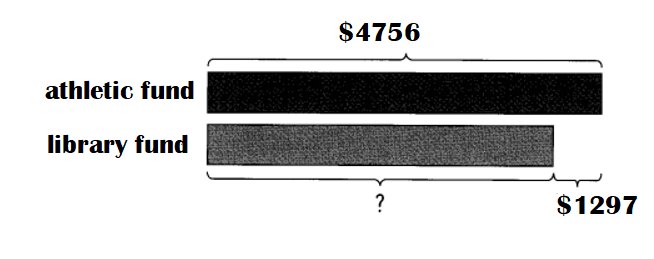Explanation:
Amount for its athletic fund a school sets = $4,756. It sets aside$1,297 less for its library fund.
=> Amount for its library fund a school sets = Amount for its athletic fund a school sets – $1297 =$4756 – $1297. =$3462.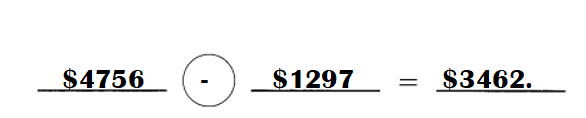b. $948 is spent from the library fund. How much money is left?$____ is left in the library fund.
Amount left for its library fund  = $2514.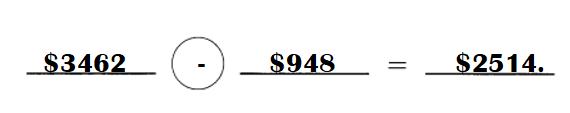Explanation: Amount for its library fund a school sets =$3462.
$948 is spent from the library fund. => Amount left for its library fund = Amount for its library fund a school sets –$948
= $3462 –$948
= \$2514.

Question 3.
The school clerk prints 635 newsletters on Monday.
She prints 96 fewer newsletters on Wednesday.

a. How many newsletters does she print on Wednesday?
Number of newsletters the school clerk prints on Wednesday = 539.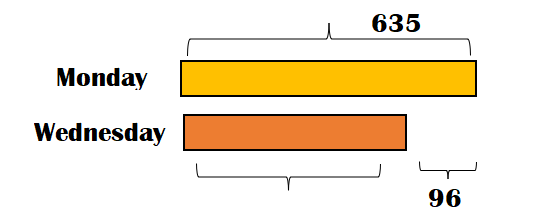Explanation:
Number of newsletters the school clerk prints on Monday = 635.
She prints 96 fewer newsletters on Wednesday.
=> Number of newsletters the school clerk prints on Wednesday = Number of newsletters the school clerk prints on Monday – 96
= 635 – 96
= 539.

b. How many newsletters does she print in all?
Total number of of newsletters the school clerk prints on Monday and Wednesday = 1174.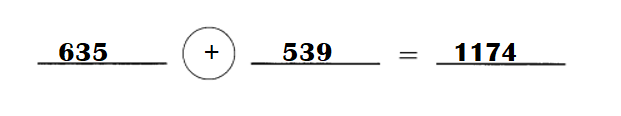Explanation:
Number of newsletters the school clerk prints on Monday = 635.
Number of newsletters the school clerk prints on Wednesday = 539.
Total number of of newsletters the school clerk prints on Monday and Wednesday = Number of newsletters the school clerk prints on Monday + Number of newsletters the school clerk prints on Wednesday
= 635 + 539
= 1174.

Question 4.
Mr. Tuzamoto’s factory makes 1,793 toys each day. It makes 157 more toys than Ms. Jefferson’s factory.

a. How many toys does Ms. Jefferson’s factory make each day?Ms. Jefferson’s factory makes ___ toys each day.
Number of toys Ms. Jefferson’s factory makes each day = 1636.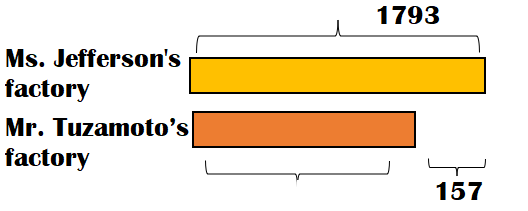Explanation:
Number of toys Mr. Tuzamoto’s factory makes each day = 1793.
It makes 157 more toys than Ms. Jefferson’s factory.
=> Number of toys Ms. Jefferson’s factory makes each day = Number of toys Mr. Tuzamoto’s factory makes each day  – 157
= 1793 – 157
= 1636.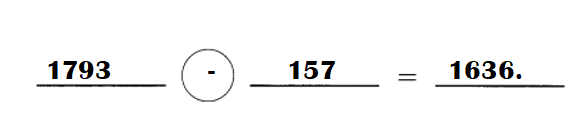b. If Ms. Jefferson’s factory sells 698 toys, how many toys does her factory have left?Ms. Jefferson’s factory has ___ toys left.
Number of toys Ms. Jefferson’s factory left over = 938.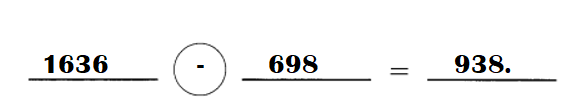Explanation:
Number of toys Ms. Jefferson’s factory makes each day = 1636.
Number of toys Ms. Jefferson’s factory sold = 698.
Number of toys Ms. Jefferson’s factory left over = Number of toys Ms. Jefferson’s factory makes each day  – 698
= 1636 – 698
= 938.

Question 5.
A middle school has 3,756 students. It has 455 fewer students than an elementary school.

a. How many students does the elementary school have?
Number of students a elementary school has = 4211.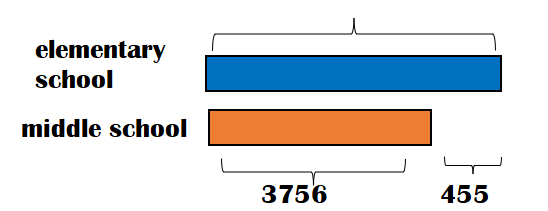Explanation:
Number of students a middle school has = 3,756.
It has 455 fewer students than an elementary school.
=> Number of students a elementary school has = Number of students a middle school has + 455
= 3756 + 455
=  4211.

b. How many students do both schools have in all?